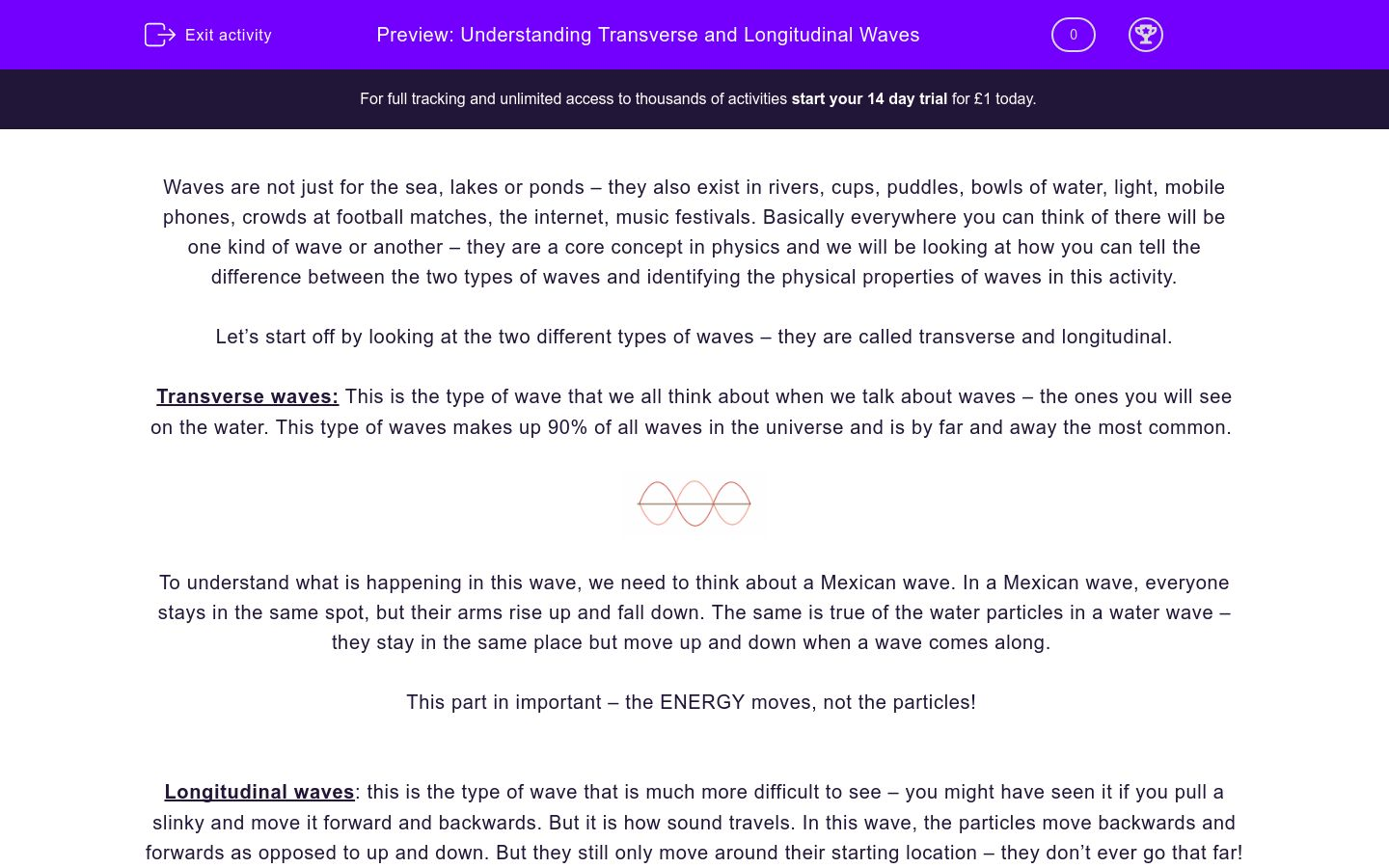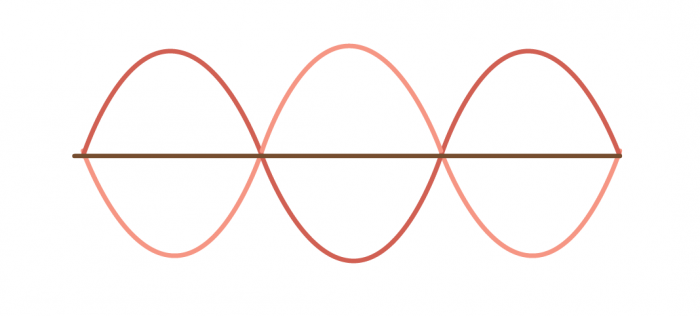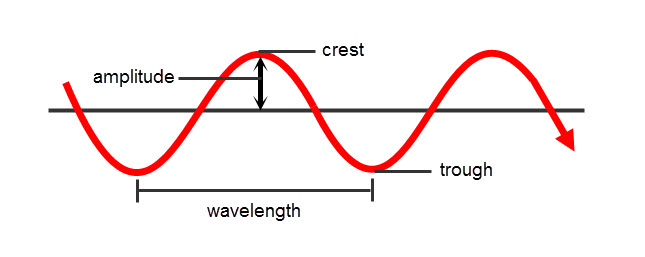# Understanding Transverse and Longitudinal Waves

In this worksheet, students will understand the difference between transverse and longitudinal waves as well as being able to describe basic wave properties like wavelength, frequency, and amplitude.Key stage:  KS 4

GCSE Boards:   AQA, AQA Trilogy, OCR 21st Century, OCR Gateway, Pearson Edexcel, Eduqas

Curriculum topic:   Waves, Radiation and Waves, Waves in Matter, Waves and Radioactivity, Waves In Matter

Curriculum subtopic:   Waves in Air, Fluids and Solids, How Do Waves Begin?, Wave Behaviour, Waves

Difficulty level:### QUESTION 1 of 10

Waves are not just for the sea, lakes or ponds – they also exist in rivers, cups, puddles, bowls of water, light, mobile phones, crowds at football matches, the internet, music festivals. Basically everywhere you can think of there will be one kind of wave or another – they are a core concept in physics and we will be looking at how you can tell the difference between the two types of waves and identifying the physical properties of waves in this activity.

Let’s start off by looking at the two different types of waves – they are called transverse and longitudinal.

Transverse waves: This is the type of wave that we all think about when we talk about waves – the ones you will see on the water. This type of waves makes up 90% of all waves in the universe and is by far and away the most common.To understand what is happening in this wave, we need to think about a Mexican wave. In a Mexican wave, everyone stays in the same spot, but their arms rise up and fall down. The same is true of the water particles in a water wave – they stay in the same place but move up and down when a wave comes along.

This part in important – the ENERGY moves, not the particles!

Longitudinal waves: this is the type of wave that is much more difficult to see – you might have seen it if you pull a slinky and move it forward and backwards. But it is how sound travels. In this wave, the particles move backwards and forwards as opposed to up and down. But they still only move around their starting location – they don’t ever go that far!This part in important – the ENERGY moves, not the particles!

Now, there are some things that we need to know about waves – the wavelength and amplitude. The amplitude is the distance from the middle of the wave to the top, or from the middle to the bottom. The wavelength is the distance of one wave (e.g. from the top of a wave to the top of the next wave).The frequency of a wave is how many waves pass a point per second and it is measured in hertz (Hz) and the time period of a wave is how long it takes for one wave to pass a point – this is measured in seconds.

We can work out the frequency from the time period by using this equation:

INSERT IMAGE OF EQUATION

It is a really simple equation, it’s just 1 divided by whatever you know. For example:

Q – Calculate the frequency of a wave with a time period of 2 s.

A:

f = 1/t
f = 1/2
f = 0.5 Hz

Simple!

Match the unit to the value.

## Column B

Time period
m
Frequency
Hz
Wavelength
s

A duck is sitting on a pond and you drop a stone into the water creating a transverse wave. Which direction will the duck move?

Up and down

Left to right

Which of the following is a longitudinal wave?

Water waves

Sound

Light

Heat

Microwaves

Describe how you would find the wavelength of a wave drawn on a piece of paper. (1 mark)In both of the above waves, what is moved from the left to the right?

What is the definition for amplitude?

The distance from the botttom of the wave to the top

The number of waves per second

The length of one wave

The distance from the top or bottom of the wave to the midde.

A wave has a time period of 0.2 s. Calculate the frequency of the wave. Give a unit for your answer.

Write down the definition of the time period of a wave? (1 mark)

A student sets up an experiment where he creates a series of wave in a water tank. He produces the waves at one end and then counts how many waves are reflected back to him at the other end. He counts 20 waves in 5 seconds. Calculate the frequency of these waves. Include the unit in your answer.

Describe what will happen the to frequency if you shorten the wavelength (2 marks)

• Question 1

Match the unit to the value.

## Column B

Time period
s
Frequency
Hz
Wavelength
m
EDDIE SAYS
Being able to match the units to the values will allow you to pick out the important information when it comes to doing equations with these values (spoiler alert - you will be looking at some more equations in the next worksheet int his sequence!). If you know that wavelength is measured in meters, then all you need to do is find where it talks about meters in order to find out the wavelength. Boom!
• Question 2

A duck is sitting on a pond and you drop a stone into the water creating a transverse wave. Which direction will the duck move?

Up and down
EDDIE SAYS
In a transverse wave, the particles move at right angles to the direction of the wave - this means that they will move up and down. If the duck is sitting on these particles, it will also move up and down. Remember - it is only the energy that is moving left to right in a transverse wave.
• Question 3

Which of the following is a longitudinal wave?

Sound
EDDIE SAYS
Light, heat, and microwaves are all different types of Electromagnetic waves (for more info on this, take a look at our worksheet 'Understanding the Electromagnetic spectrum'). These electromagnetic waves are all transverse. Water waves (as we have just said) are transverse which leaves sound waves as the only one left! Sound waves are created when the air compresses creating a longitudinal wave.
• Question 4

Describe how you would find the wavelength of a wave drawn on a piece of paper. (1 mark)

EDDIE SAYS
It's simple really, the wavelength is the amount of length one wave takes up. So, all you need to do is find one point on a wave and then follow it until you have the same point one wave later. Then just measure this! Now the important part comes in choosing your point - I would always go from the top of the wave to the top of the next wave, that way you'll never get confused. COMMON PROBLEM ALERT! Make sure you go the very VERY top of the wave to the VERY top of the next wave - not just kind of between them. You'll miss out on marks if you are not accurate with this!
• Question 5In both of the above waves, what is moved from the left to the right?

Energy
EDDIE SAYS
The energy is the only thing that moves int eh direction of the wave in both longitudinal and transverse waves. Longitudinal waves have particles that move from the left to the right AND energy that moves from the left to the right. The particles will also bounce back and always move around their equilibrium position. Transverse waves have particles that ONLY move up and down, but the energy of the wave will move from left to right. This means that the only thing that is moving from left to right in both of the waves is the energy.
• Question 6

What is the definition for amplitude?

The distance from the top or bottom of the wave to the midde.
EDDIE SAYS
Okay - the number of waves per second is the frequency, measured in Hertz (Hz), so it can't be that one. The length of one wave is the wavelength - the one name that makes sense in this whole thing - so it can't be that one. This leaves us with a problem - is it from the top to the bottom or from the top to the middle? You'll have to remember drawing it out - you would always measure from the middle to the top, or the middle to the bottom. It must be the last one!
• Question 7

A wave has a time period of 0.2 s. Calculate the frequency of the wave. Give a unit for your answer.

5Hz
5 Hz
EDDIE SAYS
Did you remember the unit? Good - did you remember to put a capital H in Hz? It's the name of a person and so it needs a capital! So how do we do it? Frequency is 1 divided by time period so like this: f = 1/t f = 1/0.2 f = 5 Hz. Simple!
• Question 8

Write down the definition of the time period of a wave? (1 mark)

EDDIE SAYS
The time period is measured in seconds, so you know that it has to be a measurement of some form of time. You know that the only thing to do with time and waves is measuring how long it takes for a wave to do something, so the logical conclusion for that would be the amount of time it takes for a wave to pass a point!
• Question 9

A student sets up an experiment where he creates a series of wave in a water tank. He produces the waves at one end and then counts how many waves are reflected back to him at the other end. He counts 20 waves in 5 seconds. Calculate the frequency of these waves. Include the unit in your answer.

4Hz
4 Hz
EDDIE SAYS
Did you remember the unit for your answer? Hz (for Hertz), with a capital H! This question was all about finding out if you know what the definition for frequency is. If you can remember this, then you shouldn't have too much of a problem with this question. Frequency is the number of waves that pass a point per second. We know we have 20 waves in 5 seconds, so how many waves did we have in 1 second? Easy - 20/5=4 4 waves per second 4 Hz
• Question 10

Describe what will happen the to frequency if you shorten the wavelength (2 marks)

EDDIE SAYS
This one will be a challenge, so don\'t worry if you don\'t get it. But you should have guessed at an answer, even if you didn\'t know what that answer was going to be. Let\'s think about what we should have said in our answer. We know that the wavelength is the distance between two peaks of a wave, right? We also know that frequency is the number of waves that pass a point every second. If we make the length of a wave shorter, then we can fit more waves in every second, right? Think about it like this, if you line up a whole bunch of lorries and get them to drive past you at 5 m/s you\'re not gonig to get very many lorries in 20 seconds. But if you do the same with Mini\'s then you will get a lot more Mini\'s, right? its the same with waves, make the waves shorter and you\'ll get a lot more of them every second - so your frequency goes up.